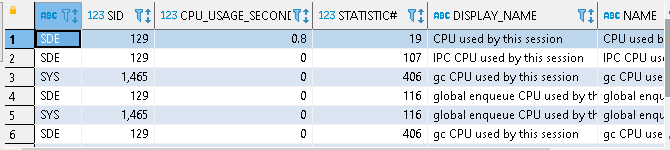# Database Software

## Understanding Oracle performance tuning query

I am having hard time in understanding oracle tuning parameters. It seem that the information is in bit and pieces.
For example, query about V\$SESSTAT, joined with other tables. Here is the query :
se.STATISTIC#, sn.DISPLAY_NAME, sn.NAME
from v\$session ss, v\$sesstat se, v\$statname sn
where se.STATISTIC# = sn.STATISTIC#
and NAME like '%CPU used by this session%'
and se.SID = ss.SID
and ss.status='ACTIVE'
order by VALUE desc; [I attached the table below]

In another query : SELECT Logon_time,
(SELECT ROUND (VALUE / 1024 / 1024, 2)
FROM v\$sesstat
WHERE STATISTIC# = 35 AND v\$sesstat.SID = v\$session.sid)
AS "PGA Memory, in MB",
(SELECT VALUE
FROM v\$sesstat
WHERE STATISTIC# = 17 AND v\$sesstat.SID = v\$session.sid)
AS "CPU, used by session",
ROUND ( (SELECT VALUE
FROM v\$sesstat
WHERE STATISTIC# = 584 AND v\$sesstat.SID = v\$session.sid)
/ (SELECT DECODE (VALUE, 0, 1, VALUE)
FROM v\$sesstat
WHERE STATISTIC# = 583 AND v\$sesstat.SID = v\$session.sid),
2)
AS "Hard Parse, %",
(SELECT ROUND (VALUE / 1024 / 1024, 2)
FROM v\$sesstat
WHERE STATISTIC# = 83 AND v\$sesstat.SID = v\$session.sid)
AS "Physical read bytes, in MB", ... [i cut the query deliberately]
In the first query, How does one know that the "se.value" has to be divided by 100 to give the result in "second" ? Whats the unit of the original "se.value" ?
In the second query, the VALUE is sometimes presented as it is and sometimes divided by 1024^2. How does one know the unit of such values ?
Where can I find a complete guide on "what is the unit of the value for what parameter" ? It seems the VALUEs have different unit for different parameters
I am using Oracle 12c
Thanks
V\$SESSTAT (0 Bytes)